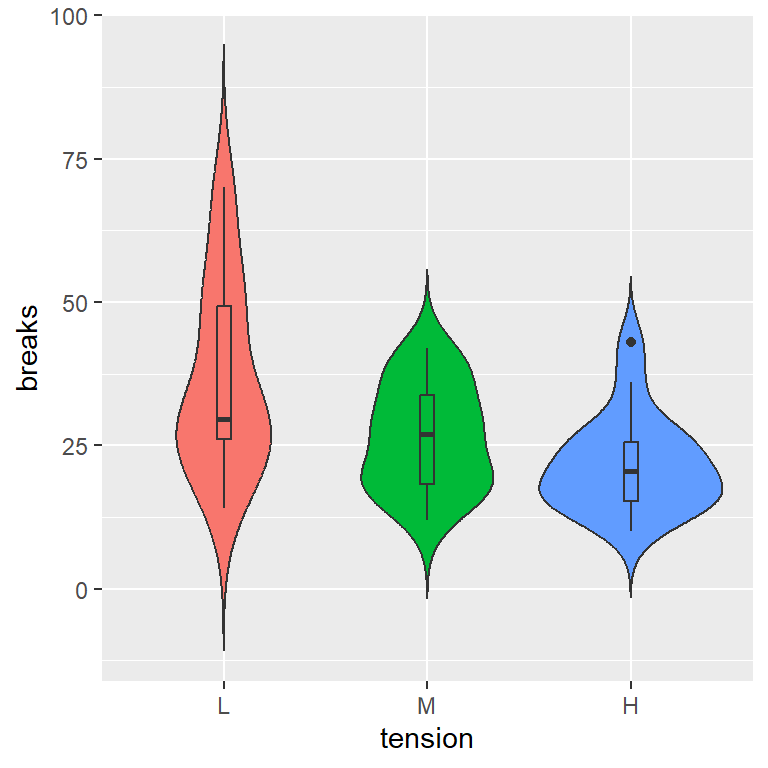# Violin plot by group in ggplot2

## Sample data

The data set used in the following examples is `warpbreaks`, which gives the number of warp breaks in a yarn, the type of wool and the level of tension.

``````# Sample data set
warpbreaks``````

## Grouped violin plot with `geom_violin`

A violin plot by group can be created in ggplot passing the numerical (`breaks`) and the categorical (`tension`) variable to `aes` and using `geom_violin`.

``````# install.packages("ggplot2")
library(ggplot2)

ggplot(warpbreaks, aes(x = tension, y = breaks)) +
geom_violin()``````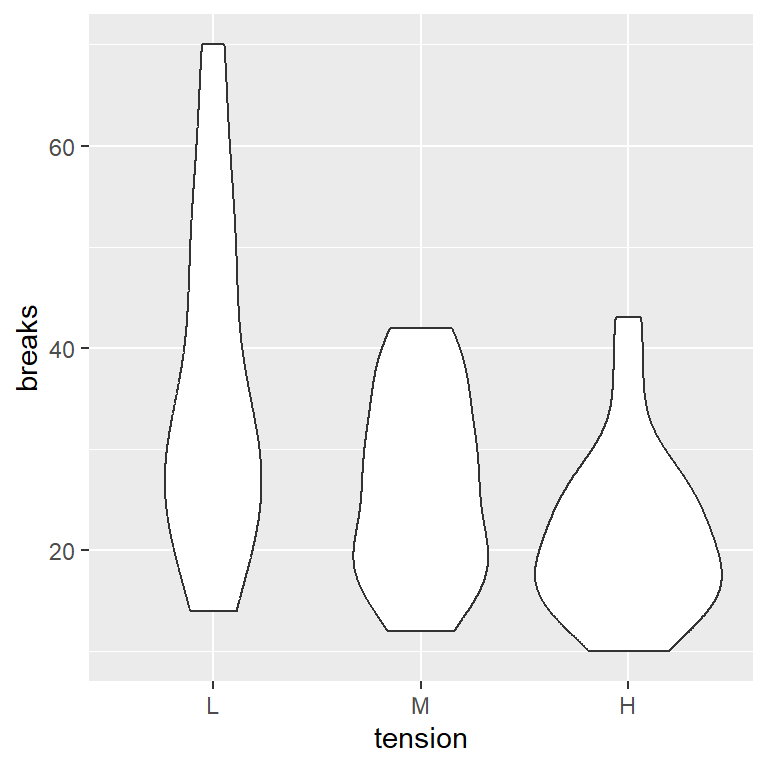Horizontal violin plot

If you want a horizontal violin plot instead of vertical you can pass the categorical variable (`tension`) to `y` or use `coord_flip` as in the example below.

``````# install.packages("ggplot2")
library(ggplot2)

ggplot(warpbreaks, aes(x = tension, y = breaks)) +
geom_violin() +
coord_flip()``````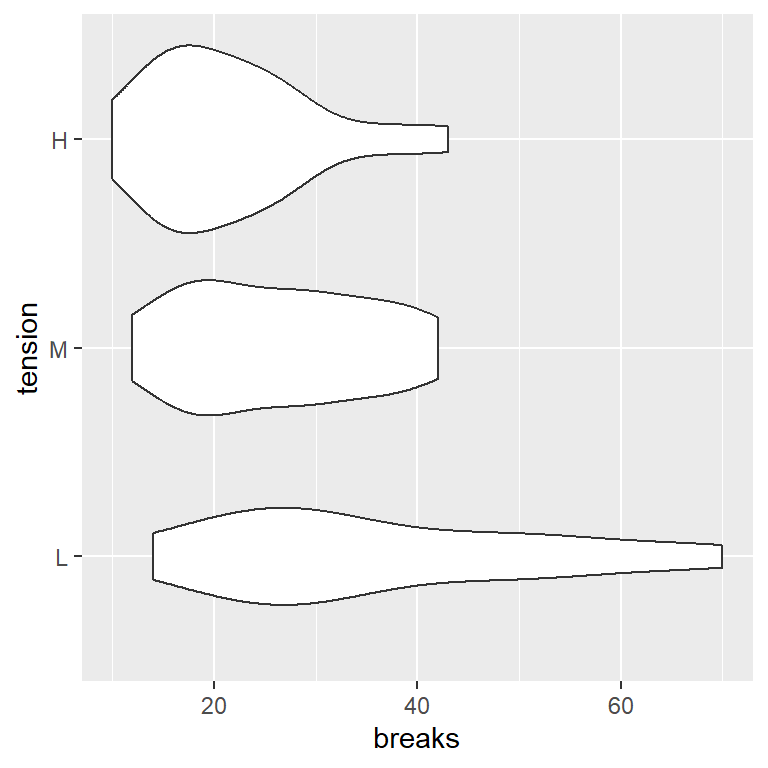Avoid trimming the trails

By default, the trails of the violin are trimmed to the range of the data. To avoid trimming set `trim = FALSE` inside `geom_violin`.

``````# install.packages("ggplot2")
library(ggplot2)

ggplot(warpbreaks, aes(x = tension, y = breaks)) +
geom_violin(trim = FALSE)``````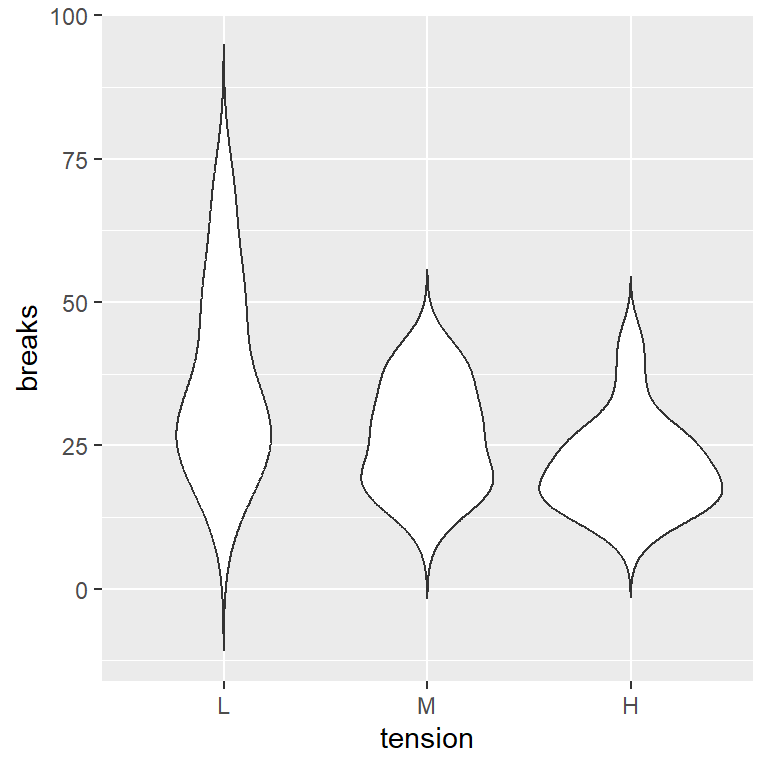The desired quantiles can be added passing a vector to the `draw_quantiles` argument, as in the example below.

``````# install.packages("ggplot2")
library(ggplot2)

ggplot(warpbreaks, aes(x = tension, y = breaks)) +
geom_violin(trim = FALSE,
draw_quantiles = c(0.25, 0.5, 0.75))``````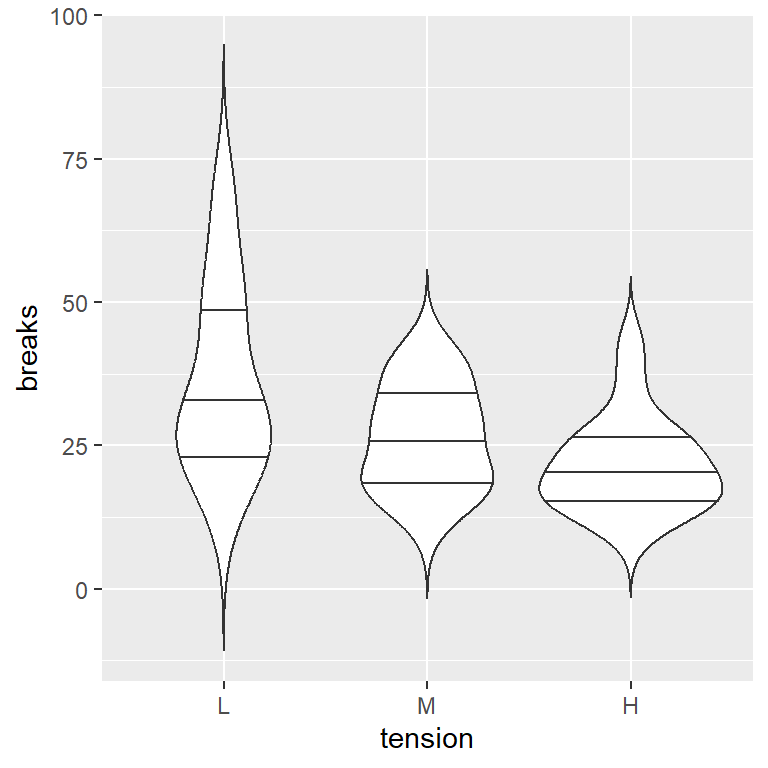You can also overlay box plots to the violin plots to show the median and the outliers. Recall to set a small width for the box plots.

``````# install.packages("ggplot2")
library(ggplot2)

ggplot(warpbreaks, aes(x = tension, y = breaks)) +
geom_violin(trim = FALSE) +
geom_boxplot(width = 0.07)``````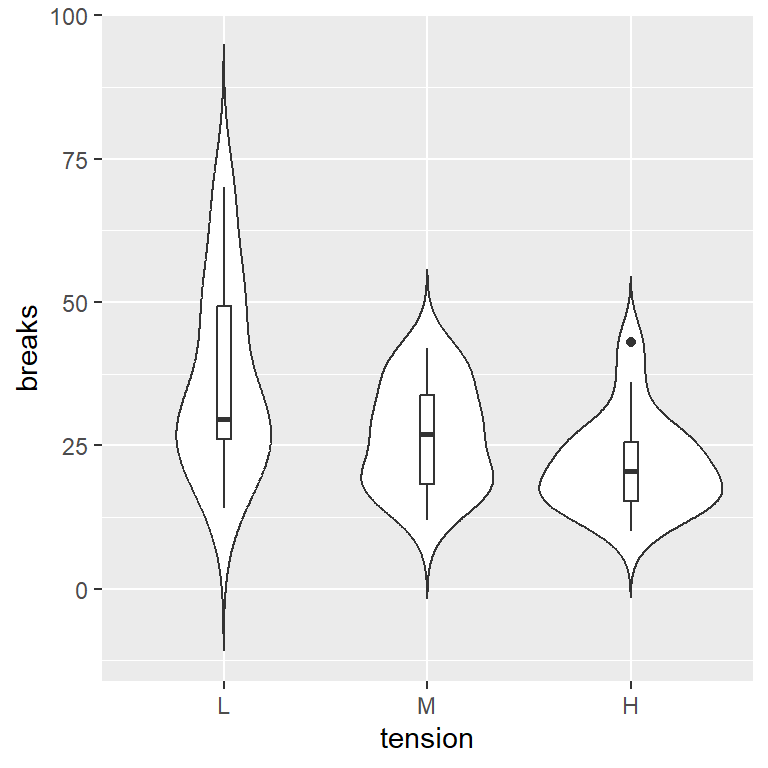Bandwidth customization

The violins are kernel density estimates of the data, so a bandwidth is involved for its computation. The default bandwidth can be changed with `bw`.

``````# install.packages("ggplot2")
library(ggplot2)

ggplot(warpbreaks, aes(x = tension, y = breaks)) +
geom_violin(trim = FALSE, bw = 10) +
geom_boxplot(width = 0.07)``````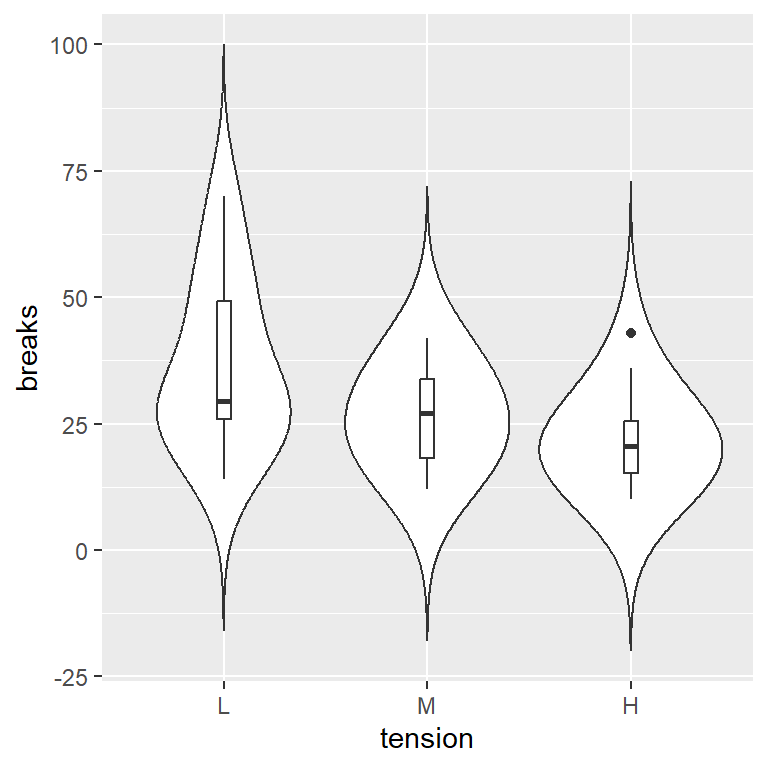## Fill and border colors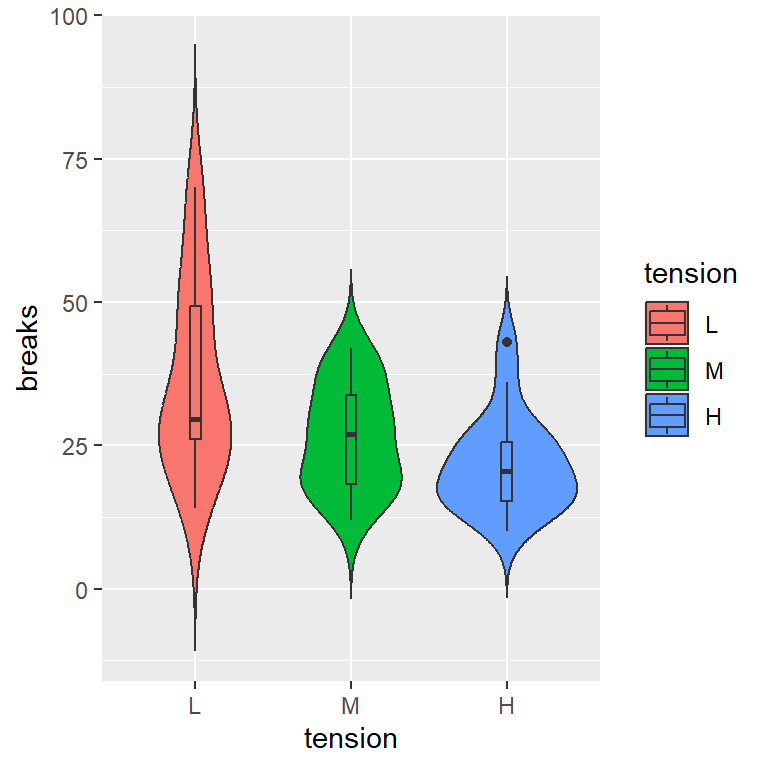Fill color by group

If you want to fill the violins by group pass the categorical variable to the `fill` argument of `aes`.

``````# install.packages("ggplot2")
library(ggplot2)

ggplot(warpbreaks, aes(x = tension, y = breaks, fill = tension)) +
geom_violin(trim = FALSE) +
geom_boxplot(width = 0.07)``````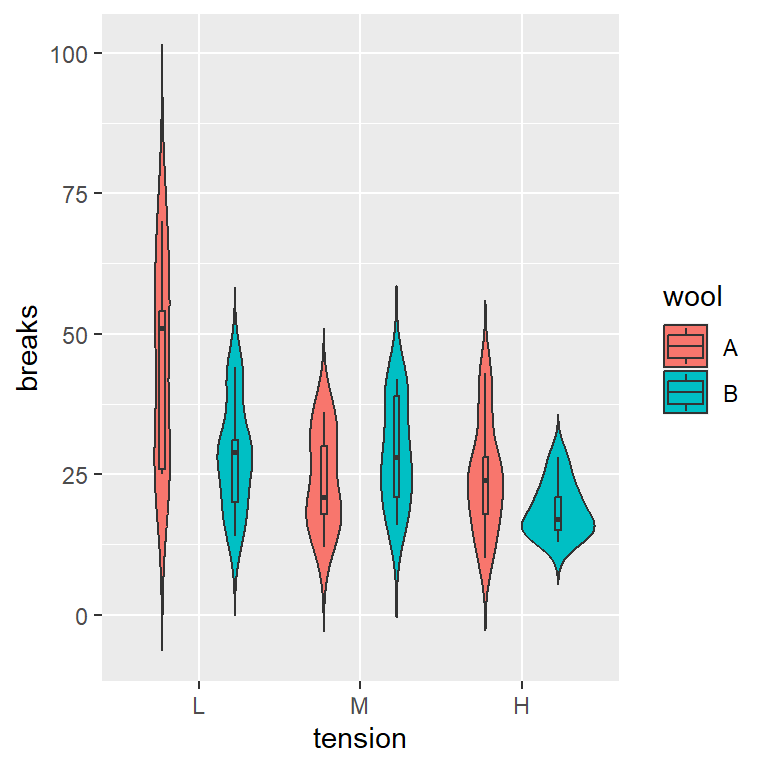Fill color by subgroup

If you have other categorical variable you can create subgroups and fill the areas based on these subgroups.

``````# install.packages("ggplot2")
library(ggplot2)

ggplot(warpbreaks, aes(x = tension, y = breaks, fill = wool)) +
geom_violin(trim = FALSE) +
geom_boxplot(width = 0.07, position = position_dodge(width = 0.9))``````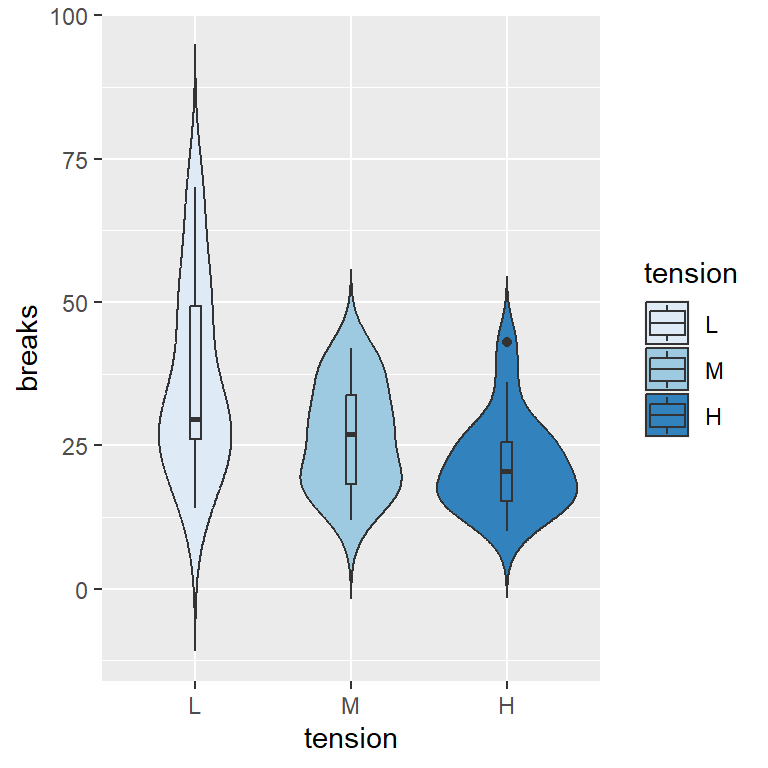Color scale

The default colors can be changed. For instance, you can use the brewer palette as follows:

``````# install.packages("ggplot2")
library(ggplot2)

ggplot(warpbreaks, aes(x = tension, y = breaks, fill = tension)) +
geom_violin(trim = FALSE) + +
geom_boxplot(width = 0.07)
scale_fill_brewer()``````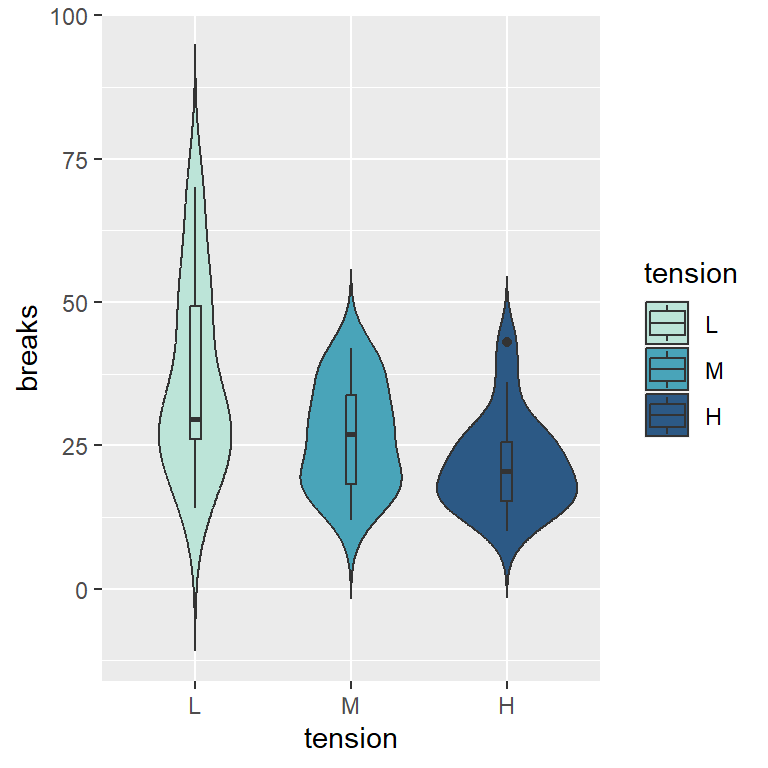Custom colors

If you want to use your custom color palette you can use `scale_fill_manual` and input the colors to the `values` argument.

``````# install.packages("ggplot2")
library(ggplot2)

ggplot(warpbreaks, aes(x = tension, y = breaks, fill = tension)) +
geom_violin(trim = FALSE) +
geom_boxplot(width = 0.07) +
scale_fill_manual(values = c("#BCE4D8", "#49A4B9", "#2C5985"))``````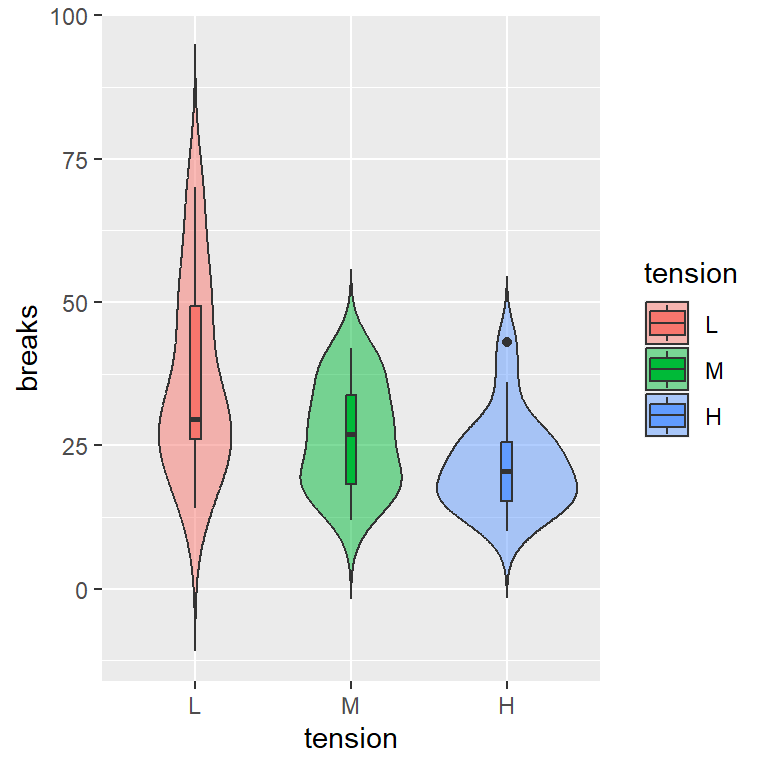Fill transparency

The fill transparency can be modified with the `alpha` argument of the `geom_violin` function.

``````# install.packages("ggplot2")
library(ggplot2)

ggplot(warpbreaks, aes(x = tension, y = breaks, fill = tension)) +
geom_violin(trim = FALSE,
alpha = 0.5) +
geom_boxplot(width = 0.07)``````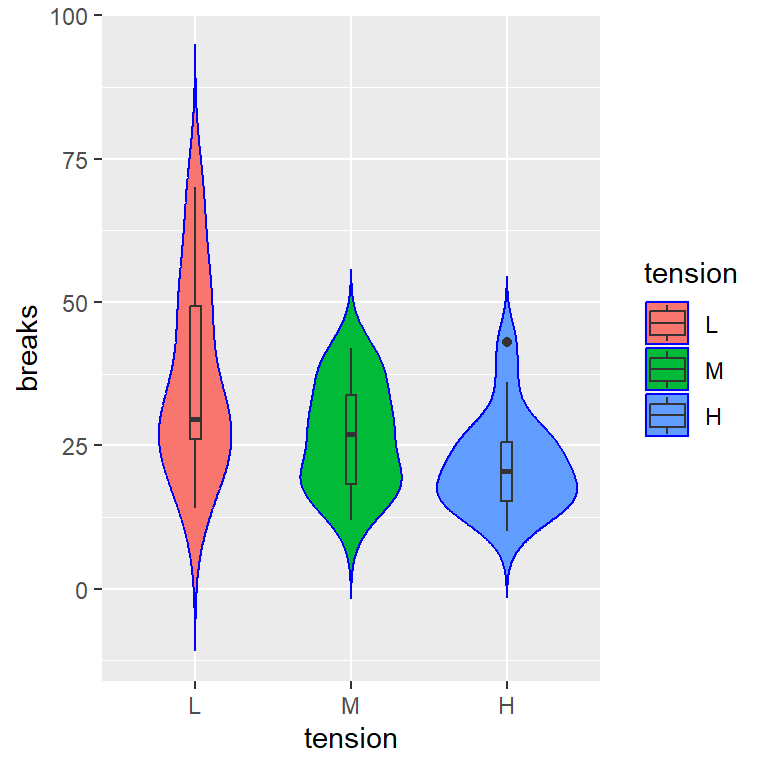Border color

The `color` argument of `geom_violin` can be used to change the color of the borders.

``````# install.packages("ggplot2")
library(ggplot2)

ggplot(warpbreaks, aes(x = tension, y = breaks, fill = tension)) +
geom_violin(trim = FALSE,
color = "blue") +
geom_boxplot(width = 0.07)``````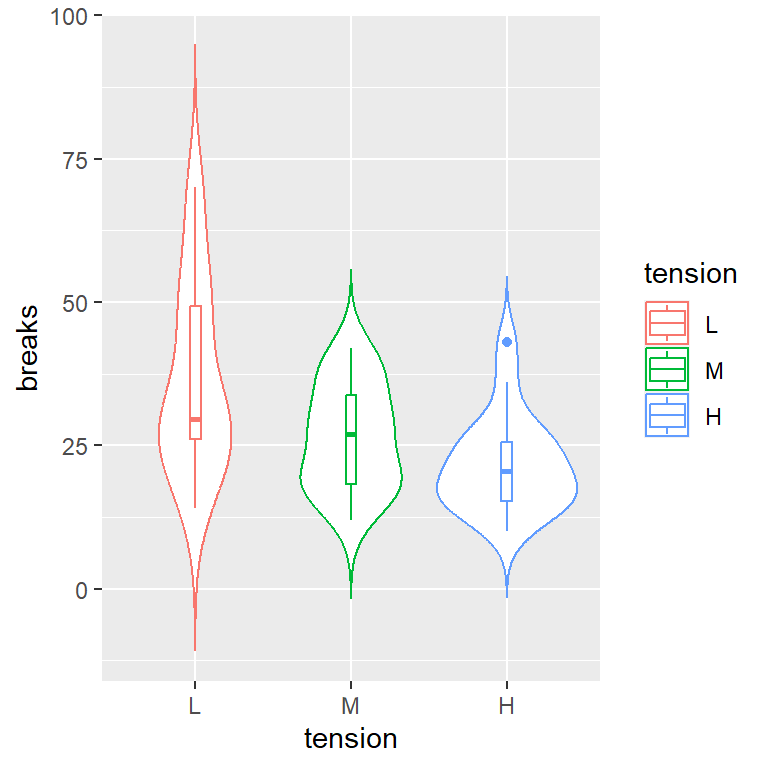Border color by group

However, if you want to set a border color based on the groups, you can pass the categorical variable to the `color` argument of the `aes` function.

``````# install.packages("ggplot2")
library(ggplot2)

ggplot(warpbreaks, aes(x = tension, y = breaks, color = tension)) +
geom_violin(trim = FALSE) +
geom_boxplot(width = 0.07)``````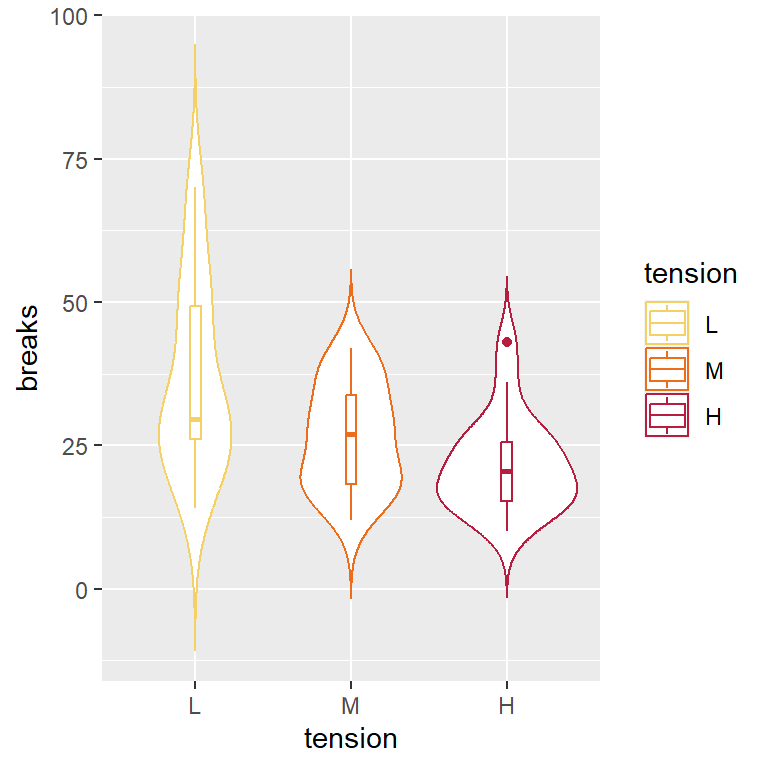Custom border colors

Similarly to changing the fill colors, you can customize the border colors, but with `scale_color_manual`.

``````# install.packages("ggplot2")
library(ggplot2)

ggplot(warpbreaks, aes(x = tension, y = breaks, color = tension)) +
geom_violin(trim = FALSE) +
geom_boxplot(width = 0.07) +
scale_color_manual(values = c("#F4D166", "#EC6E1C", "#B71D3E"))``````

## Legend customization

Legend title

The legend title displays the name of the categorical variable. To change this default title use the `guides` function as follows.

``````# install.packages("ggplot2")
library(ggplot2)

ggplot(warpbreaks, aes(x = tension, y = breaks, fill = tension)) +
geom_violin(trim = FALSE) +
geom_boxplot(width = 0.07) +
guides(fill = guide_legend(title = "Title"))``````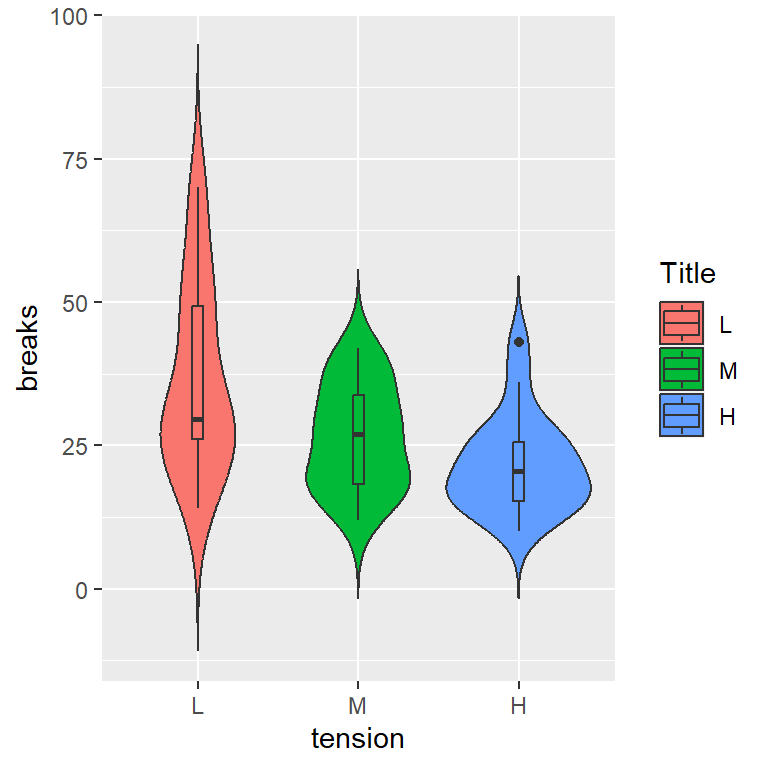Key labels

The legend key labels are the names of the groups. These labels can be changed with `scale_fill_manual` if you change the fill colors or with `scale_fill_hue` to only change the labels.

``````# install.packages("ggplot2")
library(ggplot2)

ggplot(warpbreaks, aes(x = tension, y = breaks, fill = tension)) +
geom_violin(trim = FALSE) +
geom_boxplot(width = 0.07) +
scale_fill_hue(labels = c("G1", "G2", "G3"))``````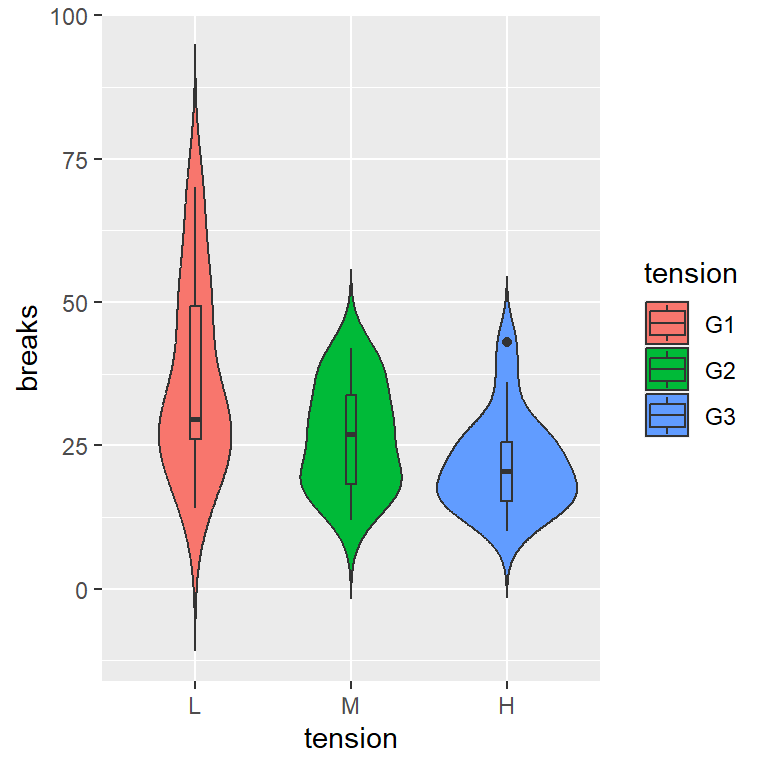Remove legend

Finally, if you want to remove the default legend you can set the legend position to `"none"` or add `show.legend = FALSE` to `geom_violin` and `geom_boxplot` (if you added it).

``````# install.packages("ggplot2")
library(ggplot2)

ggplot(warpbreaks, aes(x = tension, y = breaks, fill = tension)) +
geom_violin(trim = FALSE) +
geom_boxplot(width = 0.07) +
theme(legend.position = "none")``````